# Introduction - Relations and Functions JEE Notes | EduRev

## JEE : Introduction - Relations and Functions JEE Notes | EduRev

The document Introduction - Relations and Functions JEE Notes | EduRev is a part of the JEE Course Mathematics (Maths) Class 12.
All you need of JEE at this link: JEE

RELATION
Given any two non-empty sets A and B, A relation R from A to B is a subset of the Cartesian product A × B and is derived by describing a relationship between the first element (say x) and the other element (say y) of the ordered pairs in A & B.

Further, if (x, y) ∈ R, then we say that x is R-related to y and write this relation as x R y. Hence, R = {(x, y); x ∈ A, y ∈ B, x R Y}.

Note: Ordered pairs means (x, y) and (y, x) are two different pairs.

Consider an example of two sets, A = {2, 5, 7, 8, 9, 10, 13} and B = {1, 2, 3, 4, 5}. The Cartesian product A × B has 35 ordered pairs such as A × B = {(2, 3), (2, 5)…(13, 5)}. From this, we can obtain a subset of A × B, by introducing a relation R between the first element and the second element of the ordered pair (x, y) as
R = {(x, y): x = 4y – 3, x ∈ A and y ∈ B}
Then, R = {(5, 2), (9, 3), (13, 4)}.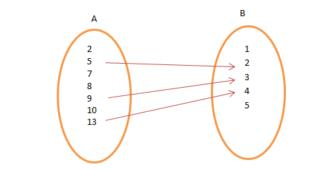(Arrow representation of the Relation R)

REPRESENTATION OF RELATION
A relation is represented either by Roster method or by Set-builder method. Consider an example of two sets A = {9, 16, 25} and B = {5, 4, 3, -3, -4, -5}. The relation is that the elements of A are the square of the elements of B.

• In set-builder form, R = {(x, y): x is the square of y, x ∈ A and y ∈ B}.
• In roster form, R = {(9, 3), (9, -3), (16, 4), (16, -4), (25, 5), (25, -5)}.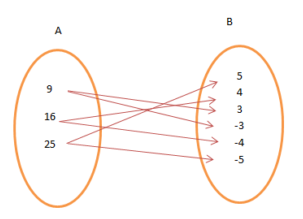TERMINOLOGIES

• Before getting into details, let us get familiar with a few terms:
• Image: Suppose we are looking in a mirror. What do we see? An image or reflection. Similarly, for any ordered pairs, in any Cartesian product (say A × B), the second element is called the image of the first element.
• Domain: The set of all first elements of the ordered pairs in a relation R from a set A to a set B. [Domain ⊆ set A]
• Range: The set of all second elements in a relation R from a set A to a set B.
• Co-domain: The whole set B. Range ⊆ Co-domain.

TOTAL NUMBER OF RELATIONS
For two non-empty set, A and B. If the number of elements in A is h i.e., n(A) = h & that of B is k i.e., n(B) = k, then the number of ordered pair in the Cartesian product will be n(A × B) = hk. The total number of relations is 2hk.

SOLVED EXAMPLE
Q.1. Let A = {5, 6, 7, 8, 9, 10} and B = {7, 8, 9, 10, 11, 13}. Define a relation R from A to B by
R = {(x, y) : y = x + 2}. Write down the domain, codomain and range of R.
Ans. Here, R = {(5, 7), (6, 8), (7, 9), (8, 10), (9, 11)}.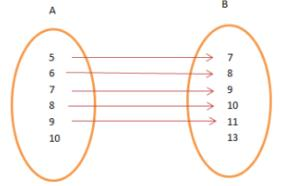Domain = {5, 6, 7, 8, 9}
Range = {7, 8, 9, 10, 11}
Co-domain = {7, 8, 9, 10, 11, 13}.

TYPES OF RELATIONS
A connection between the elements of two or more sets is Relation. The sets must be non-empty. A subset of the Cartesian product also forms a relation R. A relation may be represented either by Roster method or by Set-builder method.
Let A and B be two sets such that A = {2, 5, 7, 8, 10, 13} and B = {1, 2, 3, 4, 5}. Then,
R = {(x, y): x = 4y – 3, x ∈ A and y ∈ B} (Set-builder form)
R = {(5, 2), (10, 3), (13, 4)} (Roster form)

TYPES OF RELATIONS OR RELATIONSHIP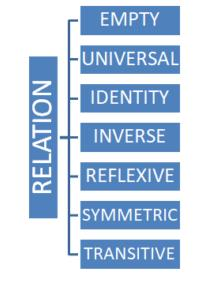(i) Empty Relation: If no element of set X is related or mapped to any element of X, then the relation R in A is an empty relation, i.e, R = Φ. Think of an example of set A consisting of only 100 hens in a poultry farm. Is there any possibility of finding a relation R of getting any elephant in the farm? No! R is a void or empty relation since there are only 100 hens and no elephant.

(ii) Universal Relation: A relation R in a set, say A is a universal relation if each element of A is related to every element of A, i.e., R = A × A. Also called Full relation. Suppose A is a set of all - natural numbers and B is a set of all whole numbers. The relation between A and B is universal as every element of A is in set B. Empty relation and Universal relation are sometimes called trivial relation.

(iii) Identity Relation: In Identity relation, every element of set A is related to itself only. I = {(a, a), ∈ A}.
For example: If we throw two dice, we get 36 possible outcomes, (1, 1), (1, 2), … , (6, 6). If we define a relation as R: {(1, 1), (2, 2), (3, 3), (4, 4), (5, 5), (6, 6)}, it is an identity relation.

(iv) Inverse Relation: Let R be a relation from set A to set B i.e., R ⊆ A × B. The relation R-1 is said to be an Inverse relation if R-1 from set B to A is denoted by R-1 = {(b, a): (a, b) ∈ R}. Considering the case of throwing of two dice if R = {(1, 2), (2, 3)}, R-1 = {(2, 1), (3, 2)}. Here, the domain of R is the range of R-1 and vice-versa.

(v) Reflexive Relation: If every element of set A maps to itself, the relation is Reflexive Relation. For every a ∈ A, (a, a) ∈ R.
For example: Let A = {1, 2, 3}
Then R1 = {(1, 1), (2, 2), (3, 3)}
R2 = {(1, 1), (2, 2), (3, 3), (1, 2)}
R3 = {(1, 1), (2, 2), (1, 2)}
Clearly ,R1, R2 both are reflexive relation, but R3 is not reflexive relation because (3, 3) ∉ R3
Note: Every identity relation is reflexive relation,but every reflexive relation is not identity relation.

(vi) Symmetric Relation: A relation R on a set A is said to be symmetric if (a, b) ∈ R then (b, a) ∈ R, a & b ∈ A.
For example: Let A = {1, 2, 3}
Then R1 = {(1, 1), (2, 2), (3, 3)}
R2 = {(1, 1), (2, 2), (3, 3), (1, 2), (2, 1)}
R3 = {(1, 1), (2, 2), (1, 2)}
Clearly, R1, R2 both are symmetric relation, but R3 is not symmetric relation because (1, 2) ∈ R3 , but (2, 1) ∉ R3
Note: A relation is called symmetric if R = R-1.

(vii) Transitive Relation: A relation in a set A is transitive if, (a, b) ∈ R, (b, c) ∈ R, then (a, c) ∈ R, a, b, c ∈ A
For example: Let A = {1, 2, 3}
Then R1 = {(1, 1), (2, 2), (3, 3)}
R2 = {(1, 1), (2, 2), (3, 3), (1, 2), (2, 1)}
R3 = {(1, 1), (2, 2), (1, 2), (2, 3)}
Clearly ,R1, R2 both are transitive relation, but R3 is not transitive relation because(1, 2) & (2, 3) ∈ R3, but (1, 3) ∉ R3

Note

• Every empty relation  defined on a non empty set is always symmetric and transitive but not reflexive.
• Every universal set defined on non empty set is always reflexive, symmetric and transitive.

(viii) Equivalence Relation: A relation is said to be equivalence if and only if it is Reflexive, Symmetric, and Transitive.
For example: If we throw two dices A & B and note down all the possible outcome.

Define a relation R= {(a, b): a ∈ A, b ∈ B}, we find that {(1, 1), (2, 2), …, (6, 6) ∈ R} (reflexive). If {(a, b) = (1, 2) ∈ R} then, {(b, a) = (2, 1) ∈ R} (symmetry). If {(a, b) = (1, 2) ∈ R} and {(b, c) = (2, 3) ∈ R} then {(a, c) = (1, 3) ∈ R} (transitive)

SOLVED EXAMPLE
Q.1. If A is a set of all triangles and the relation R is defined by “is congruent to” prove that R is an equivalence relation.
Ans.
(i) R is reflexives as every triangle is congruent to itself.
(ii) R is symmetric: if a triangle x is congruent to another triangle y, then the triangle y is congruent to the triangle x.
(iii) If ‘a triangle x is congruent to a triangle y and y is congruent to a third triangle z, then x is also congruent to z.
Hence the relation R is transitive. (i), (ii) and (iii) ⇒ that the relation R is an equivalence relation.

Q.2. Three friends A, B, and C live near each other at a distance of 5 km from one another. We define a relation R between the distances of their houses. Is R an equivalence relation?
Ans. For an equivalence Relation, R must be reflexive, symmetric and transitive.

• R is not reflexive as A cannot be 5 km away to itself.
• The relation, R is symmetric as the distance between A & B is 5 km which is the same as the distance between B & A.
• R is transitive as the distance between A & B is 5 km, the distance between B & C is 5 km and the distance between A & C is also 5 km.

Therefore, this relation is not equivalent.

Equivalence class
An equivalence class is subset of a given set in which each element is related with each other. Moreover equivalence class can be found if the given relation on given set is an equivalence relation.

Example.
Let A = {1, 3, 5, 9, 11, 18} and a relation on A given by
R = {(a, b) | a - b is divisible by 4 } find all equivalence classes.

Solution. clearly R ={(1, 1), (3, 3), (5, 5), (9, 9), (11, 11), (18, 18), (1, 5)(5, 1), (1, 9), (3, 11)(11, 3), (5, 9), (9, 5)}
Now A= {1, 5, 9}, A2 = {3, 11}, A3= {18}
Hence A1, A2, A3 are called equivalence classes of a set A on the given relation R
Moreover we can represent these classes in a very specific way as below:
A1 =  or  or 
A2 =  or 
A= 
Clearly, A1, A2 , A3  are disjoint and A1∪ A2 ∪ A3 = A  and A∩ A∩ A3= ∅

Note:

• All elements of Ai are related to each other, for all i.
• No element of Ai is related to any element of Aj, i≠ j.
• ∪ Aj = X and Ai ∩Aj = ∅, i ≠ j.

The subsets Ai are called equivalence classes and are called partitions or subdivisions of set A and these are mutually disjoint to one another.

FUNCTIONS
A function is a relation that maps each element x of a set A with one and only one element y of another set B. In other words, it is a relation between a set of inputs and a set of outputs in which each input is related with a unique output. A function is a rule that relates an input to exactly one output.It is a special type of relation. A relation f from a set A to a set B is said to be a function if every element of set A has one and only one image in set B and no two distinct elements of B have the same mapped first element.  A and B are the non-empty sets. The whole set A is the domain and the whole set B is codomain.

Definition: A function is a rule (or a set of rules) which relates or associates each and every element of a non empty set A with the unique element of the non empty set B

REPRESENTATION
A function f: X → Y is represented as f(x) = y, where, (x, y) ∈ f and x ∈ X and y ∈ Y.

For any function f, the notation f(x) is read as “f of x” and represents the value of y when x is replaced by the number or expression inside the parenthesis. The element y is the image of x under f and x is the pre-image of y under f.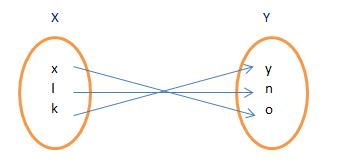Every element of the set has an image which is unique and distinct. If we notice around, we can find many examples of functions.

If we lift our hand upward, it is a function. Waving our hand freely, it is a function. A walk in a circular track, yes, it is a type of function. Now you can think of other examples too! A graph can represent a function. The graph is the set of all pairs of the Cartesian product.

Does this mean that every curve in the world defines a function? No, not every curve drawn is a function. How to find it? Vertical line test. If any curve intercepts a vertical line at more than one point, it is a curve only not a function.

Note: Every function is relation but every relation need not be a function.
For example: Let A = {1, 2, 3}
R1 = {(1, 1), (2, 3), (3, 3)}
R2 = {(1, 1)(1, 2), (3, 2)(2, 1)}
Here R1 is a function but R2 is not a function because here element 1 has two images as 1 and 2.

Q.3. Which of the following is a function?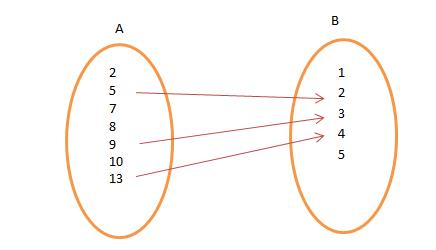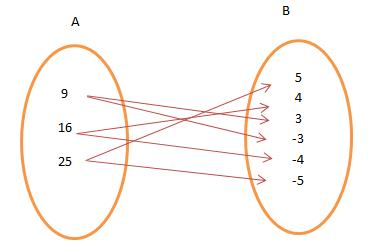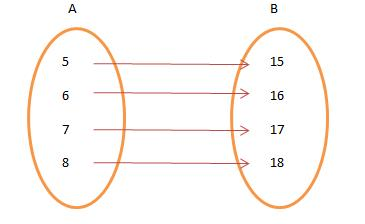Ans. Figure 3 is an example of function since every element of A is mapped to a unique element of B and no two distinct elements of B have the same pre-image in A.

Q.1. Show that the number of equivalence relation in the set {1, 2, 3} containing (1, 2) and (2, 1) is two.
Ans. A = {1, 2, 3}
The maximum possible relation (i.e. universal relation) is
R = {(1, 1), (2, 2), (3, 3), (1, 2), (1, 3), (2, 1), (2, 3), (3, 1), (3, 2)}
The smallest equivalence relation R.1 containing (1 , 2) and (2 , 1) is
R.1 = {(1, 1), (2, 2), (3, 3), (1, 2), (2, 1)}
we are left with four pairs (from universal relation) i.e. (2, 3), (3, 2), (1, 3) and (3, 1)
If we add (2, 3) to R1, then for symmetric by we must add (3, 2) and now for transitivity we are forced to add (1, 3) and (3, 1)
Thus the only relation bigger than R1 is universal relation i.e R
∴ The no. of equivalence relations containing (1, 2) and (2, 1) is two.

Q.2. If 𝑅 = {(𝑥, 𝑦) ∶ 𝑥2 + 𝑦2 ≤ 4 ; 𝑥, 𝑦 ∈ 𝑧} is a relation on 𝑧. Write the domain of R.
Ans. R = {(0, 1), (0, -1), (0, 2), (0, -2), (1, 1), (1, -1), (-1, 0), (-1, 1), (-1, -1), (2, 0), (-2, 0)}
∴ Domain of R = {0, 1 , -1, 2, -2}
(i.e the first domain of each ordered pairs)

Q.3. Let R = {(𝑥, 𝑦) : ∣𝑥2 − 𝑦2∣ < 1} be a relation on set A = {1, 2, 3, 4, 5}. Write R as a set of ordered pairs.
Ans. A = {1, 2, 3, 4, 5}
for ∣𝑥2 − 𝑦2∣ < 1 : 𝑥 should be equal to 𝑦
∴ R = {(1, 1), (2, 2), (3, 3), (4, 4), (5, 5)}

Q.4. R is a relation in Z defined as (𝑎, 𝑏) ∈ 𝑅 ⇔ 𝑎2 + 𝑏2 = 25. Find the range.
Ans. We have, 𝑎2 + 𝑏2  = 25 and 𝑎 , 𝑏 ∈ 𝑧
∴ R = {(0, 5), (0, -5), (3, 4), (3, -4), (-3, 4), (-3, -4), (4, 3), (4, -3), (-4, 3), (-4, -3), (5, 0), (-5, 0)}
∴ Range = {-5, 5, 4, -4, 4, 3, -3, 0}
(i.e. second elements of each order pairs)

Offer running on EduRev: Apply code STAYHOME200 to get INR 200 off on our premium plan EduRev Infinity!

## Mathematics (Maths) Class 12

209 videos|222 docs|124 tests

,

,

,

,

,

,

,

,

,

,

,

,

,

,

,

,

,

,

,

,

,

;